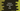# How to decrement a for loop in Python## How to decrement a for loop in Python:

for loops are used for iterating. In most cases, we uses loops in increment order. But, we can also use for loops in decrement order. In this post, we will learn how to use a decrement for loop in Python.

## Method 1: By using range() function:

range function is used to create a range of numbers in a given start and end value. range is defined as like below:

``range(start, stop, step)``

Here,

• start is an optional value. This is a number defining the start position. By default, it is 0.
• stop is the value where to stop the range. It is not included in the range.
• step is another optional value. It defines the number of steps to increment or decrement while iterating. By default, it is 1.

Let’s take a look at the below example:

``````for v in range(5):
print(v)``````

It will print:

``````0
1
2
3
4``````

We have added only stop value here, without start and step. So, it starts at 0 and ends at 4 with step value as 1.

Now, let’s try another example with all values:

``````for v in range(10, 20, 2):
print(v)``````

It starts from 10, ends at 20 and step is 2. It will print the below output:

``````10
12
14
16
18``````

### Using range() to decrement the index value:

We can change the step value to a negative value to decrement the values. For example, let me change the above example to decrement:

``````for v in range(18, 9, -2):
print(v)``````

It will start at 18 and ends at 9 with step level -2. It will print:

``````18
16
14
12
10``````

It starts at 18, decrement 2 on each step and before 9 is reached it stops, i.e. it stops at 10.

## Method 2: By using reversed() method:

If you don’t want to use step with a negative value, we can also use the reversed method and pass the range() output to this method. reversed method takes one sequence as the parameter and returns the reversed iterator for the sequence.

If we use reversed(), we don’t have to use step. But step with a negative value is preferred as reversed() adds one extra processing to the range.

``````for v in reversed(range(8)):
print(v)``````

It will print:

``````7
6
5
4
3
2
1
0``````

Another disadvantage of using reversed is that we can’t add a gap between the numbers without using a step in the range.